# Problems of the Week

Contribute a problem

# 2017-12-04 Intermediate

When sunlight is passed through a prism, it splits into a spectrum of different wavelengths $\lambda$ (different colors). On careful examination, we notice some missing bands, which indicate the presence of different elements such as hydrogen, sodium, and magnesium that absorb light of specific wavelengths.

The spectrum of a distant star, Hayesmark, which is known to have the same elemental composition as the Sun, was measured, and the data is shown below.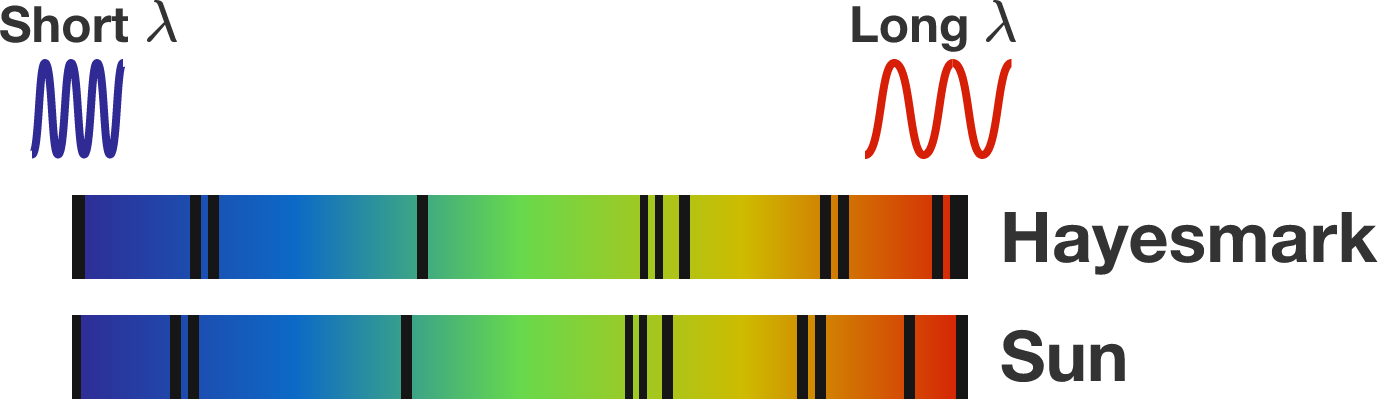What can we infer from the spectra?

Hint: The Doppler effect tells us how the wavelength measured by an observer depends on their relative velocity, $v,$ with the source. It changes according to $\frac{\lambda_o}{\lambda_s} = \sqrt{\frac{c + v}{c - v}},$ where $c$ is the speed of light.

This star is named in honor of Mark Hayes, who pointed out a problem with the star we originally chose.

Find the largest possible integer value of $x$ such that the expression below is also an integer:

$\sqrt{x^2+9x}.$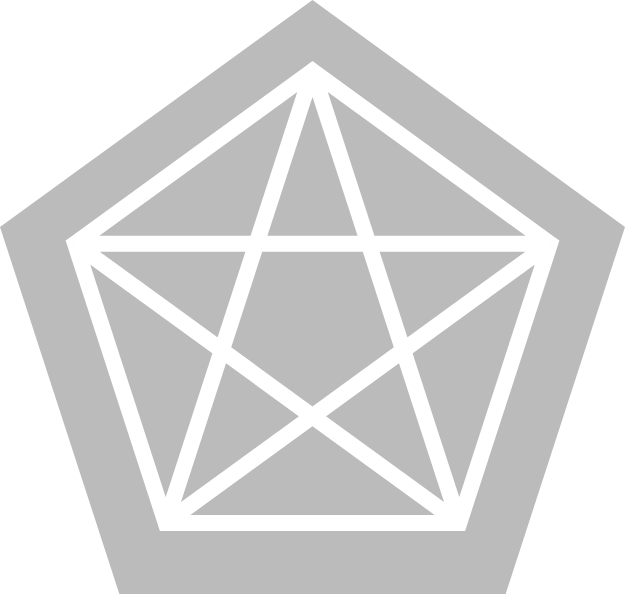CJ and Dan are playing a game in which they take turns coloring the sides and diagonals of a regular pentagon, shown in white to the right. CJ uses a blue marker while Dan uses a red marker. A player can only color the entire segment between two vertices of the pentagon, and cannot color a segment that has already been colored. The first player to make a triangle entirely of their own color for which all three vertices are vertices of the pentagon wins the game.

If CJ goes first and both players play optimally, who will win the game?

Brilli and Ignor the ants were playing on the edge of a cliff when Ignor accidentally knocked a stick off the edge and started to fall with it. Fortunately, Brilli managed to lasso a string onto the end of the stick, and is now holding on with all his might to save Ignor.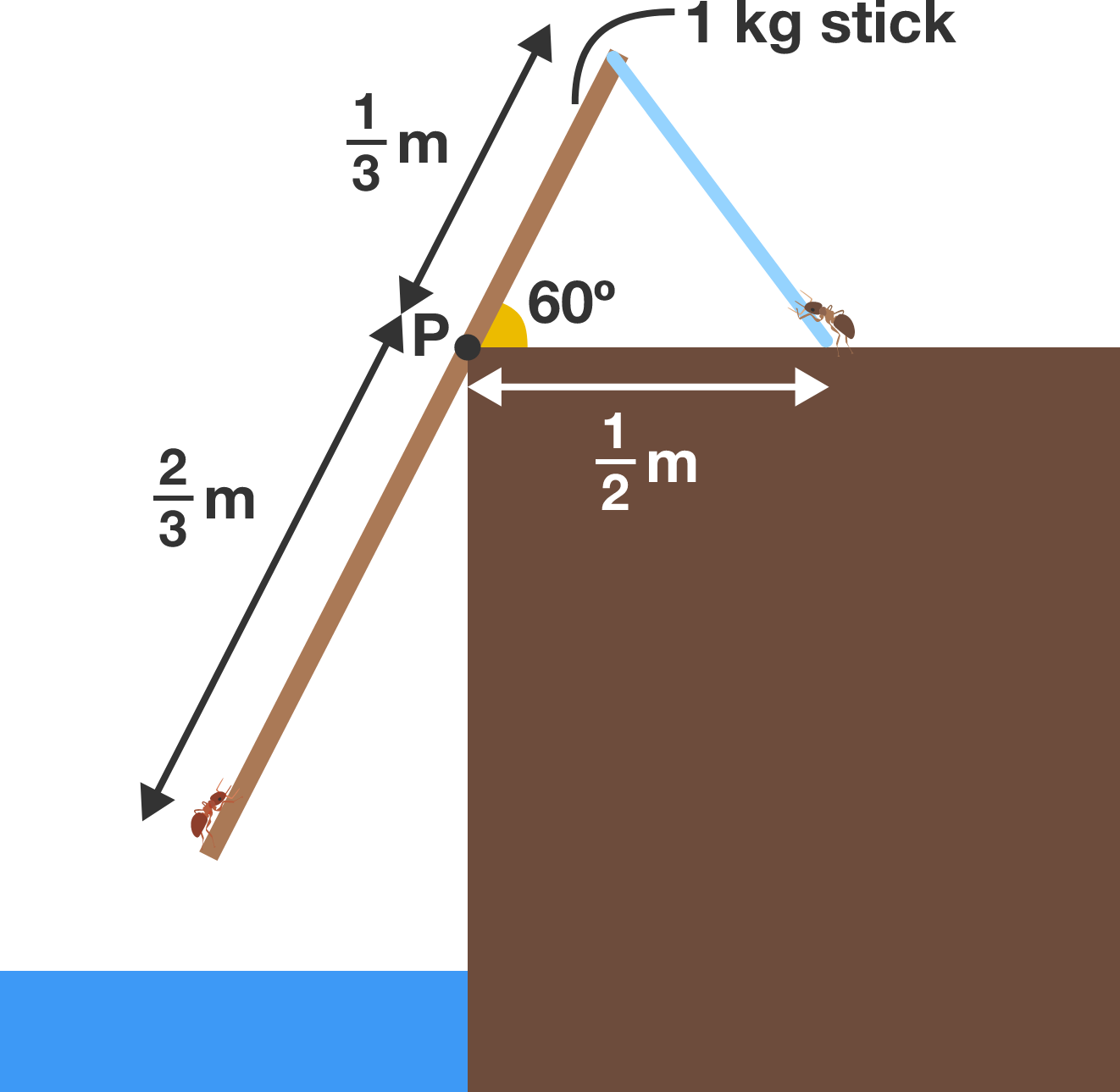A small knot at point $P$ keeps the stick from sliding along the cliff edge. Both Brilli and the string are strong enough to hold this state indefinitely, but Brilli worries that the stick might break. What is the magnitude of the net reaction force on the stick at point $P$?

Details and Assumptions:

• The mass of the stick is uniform.
• Assume the mass of the stick is much greater than the masses of the ants.
• There is an ambient downward gravitational acceleration of $10 \text{ m/s}^2.$
• The knot at point $P$ behaves like a hinge.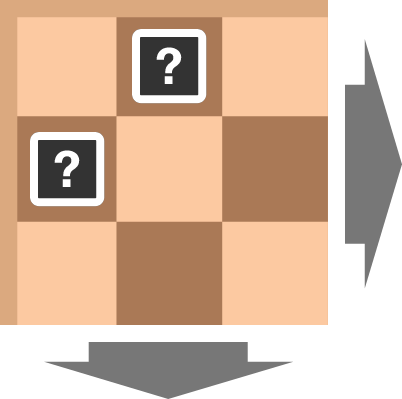On a chessboard of a finite size, we want to place kings and knights such that each king attacks exactly 2 kings and 2 knights and each knight attacks exactly 2 kings and 2 knights.

What can we say about the square adjacent to a corner (horizontally or vertically, not diagonally)?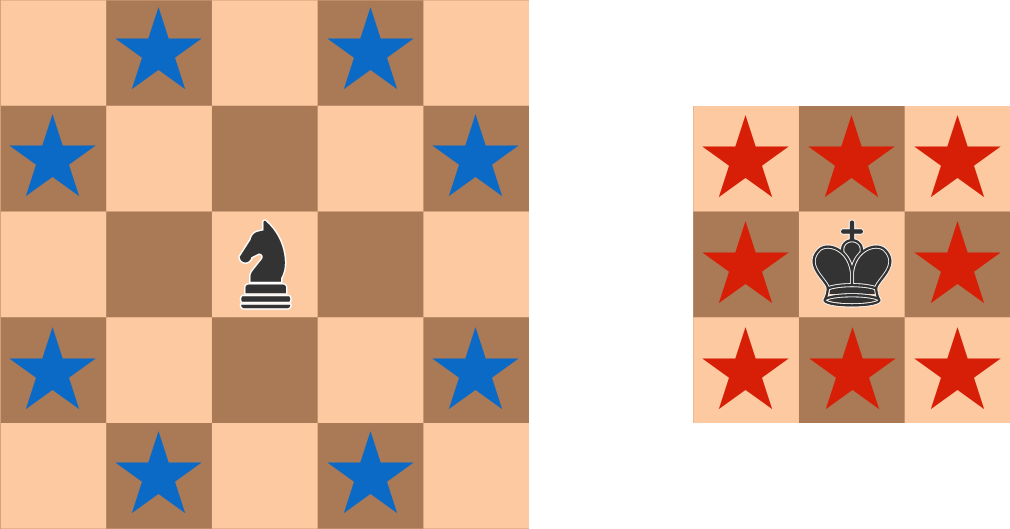The possible attacks of a knight are shown on the left, and the possible attacks of a king are shown on the right.

×

Problem Loading...

Note Loading...

Set Loading...